• waffle可以使用该pywaffle软件包创建该图表，并用于显示较大人群中各组的组成。 #! pip install pywaffle # Reference: ...
• python+pyecharts生成网页可视化图表，同一张网页生成多个图标
• ## python可视化图表生成（一）

千次阅读 多人点赞 2021-04-27 21:56:27
plt.show()#生成图表 三、散点图 import numpy as np import matplotlib.pyplot as plt x = np.arange(0., 5., 0.2)#从0-5之间，产生等差数列，公差为0.2 plt.plot(x, x, "r--", x, x**2, "bs", x, x**3, "g^")# plt...
一、安装拓展包
pip install matplotlib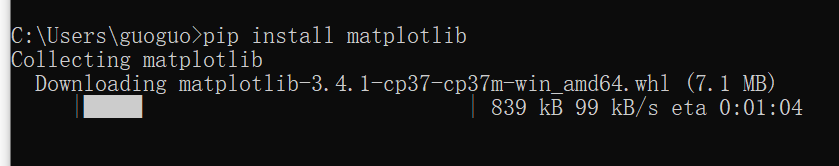二、折线图
import numpy as np
import matplotlib.pyplot as plt

x = np.linspace(0, 2, 100)#创建等差数列 0-2之间100个

plt.plot(x, x, label="line1")#第一个参数为横坐标 第二个为纵坐标 第三个为曲线名字
plt.plot(x, x**2, label="line2")
plt.plot(x, x**3, label="line3")

plt.xlabel("x label")#x轴名字
plt.ylabel("y label")#y轴名字

plt.title("测试折线图")#图标名字

plt.legend()#显示图例

plt.show()#生成图表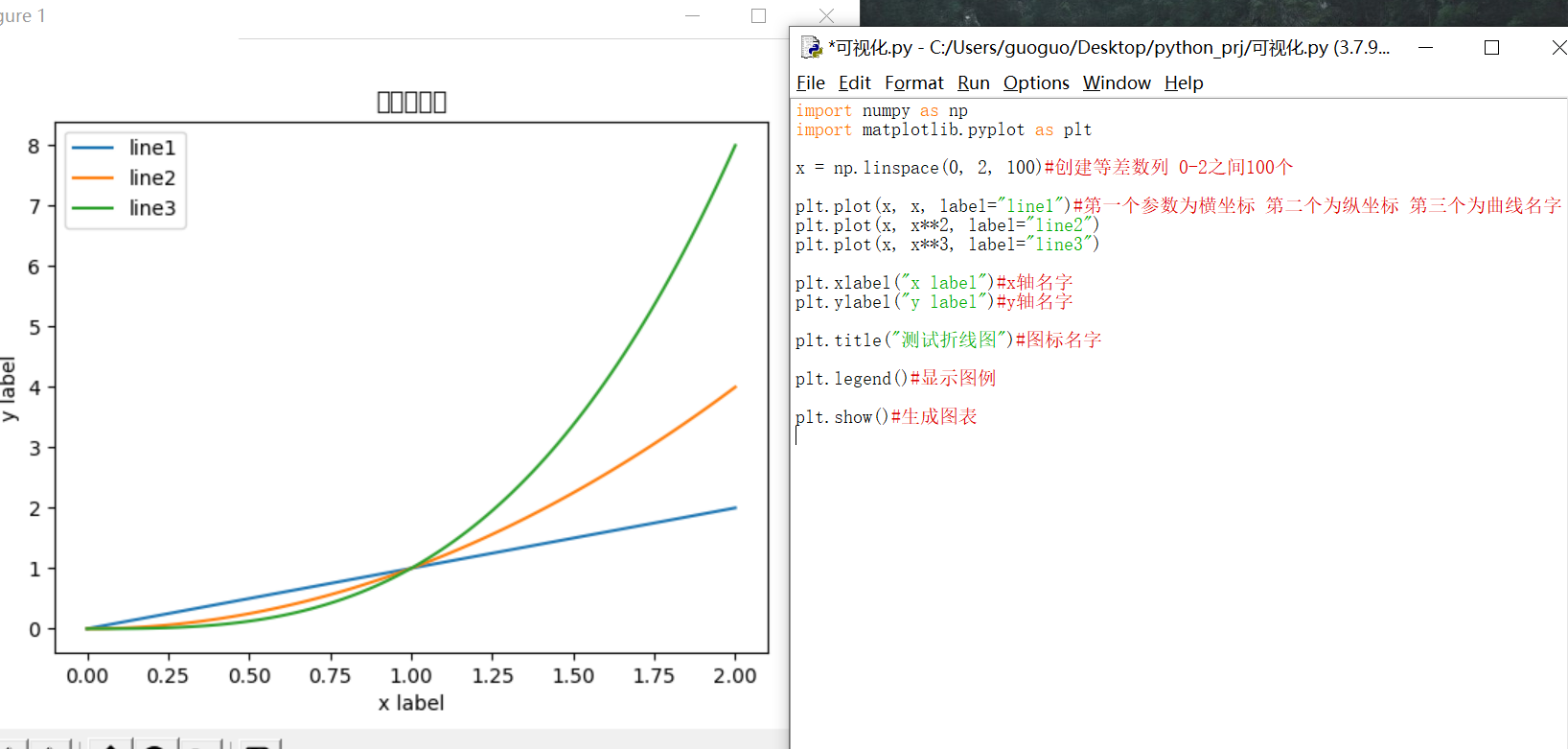三、散点图
import numpy as np
import matplotlib.pyplot as plt

x = np.arange(0., 5., 0.2)#从0-5之间，产生等差数列，公差为0.2

plt.plot(x, x, "r--", x, x**2, "bs", x, x**3, "g^")#
plt.xlabel("x label")#x轴名字
plt.ylabel("y label")#y轴名字

plt.title("测试折线图")#图标名字

plt.legend()#显示图例

plt.show()#生成图表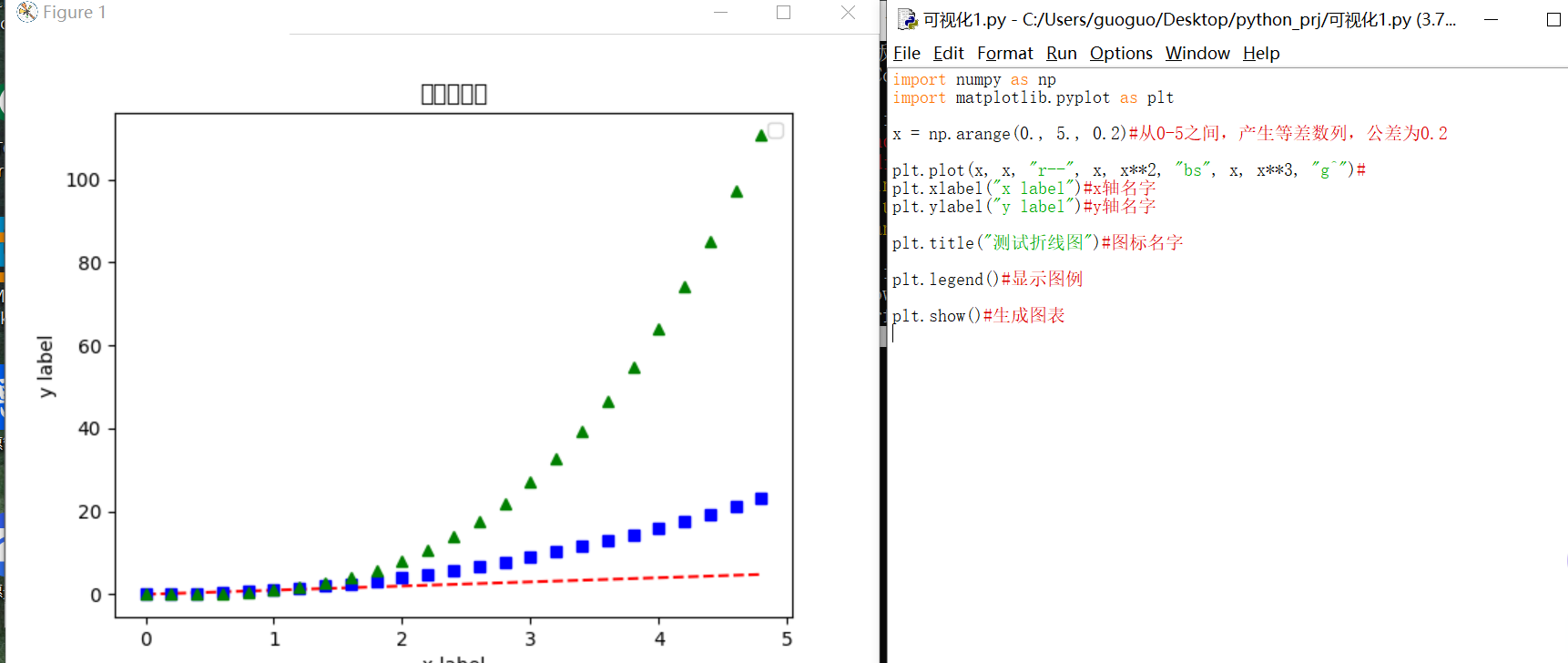四、三维散点图
import numpy as np
import matplotlib.pyplot as plt
from mpl_toolkits.mplot3d import Axes3D

data = np.random.randint(0, 255, size=[40,40,40])#产生0~255的随机数40x3个

x,y,z = data,data,data#x y z分别40个随机数
ax = plt.subplot(111, projection="3d")#创建三维绘图

ax.scatter(x[:10], y[:10], z[:10], c="y")#绘制散点
ax.scatter(x[10:20], y[10:20], z[10:20], c="r")
ax.scatter(x[30:40], y[30:40], z[30:40], c="g")

ax.set_xlabel("X")#x轴名字
ax.set_ylabel("Y")#y轴名字
ax.set_zlabel("Z")#z轴名字

plt.title("测试折线图")#图标名字

plt.legend()#显示图例

plt.show()#生成图表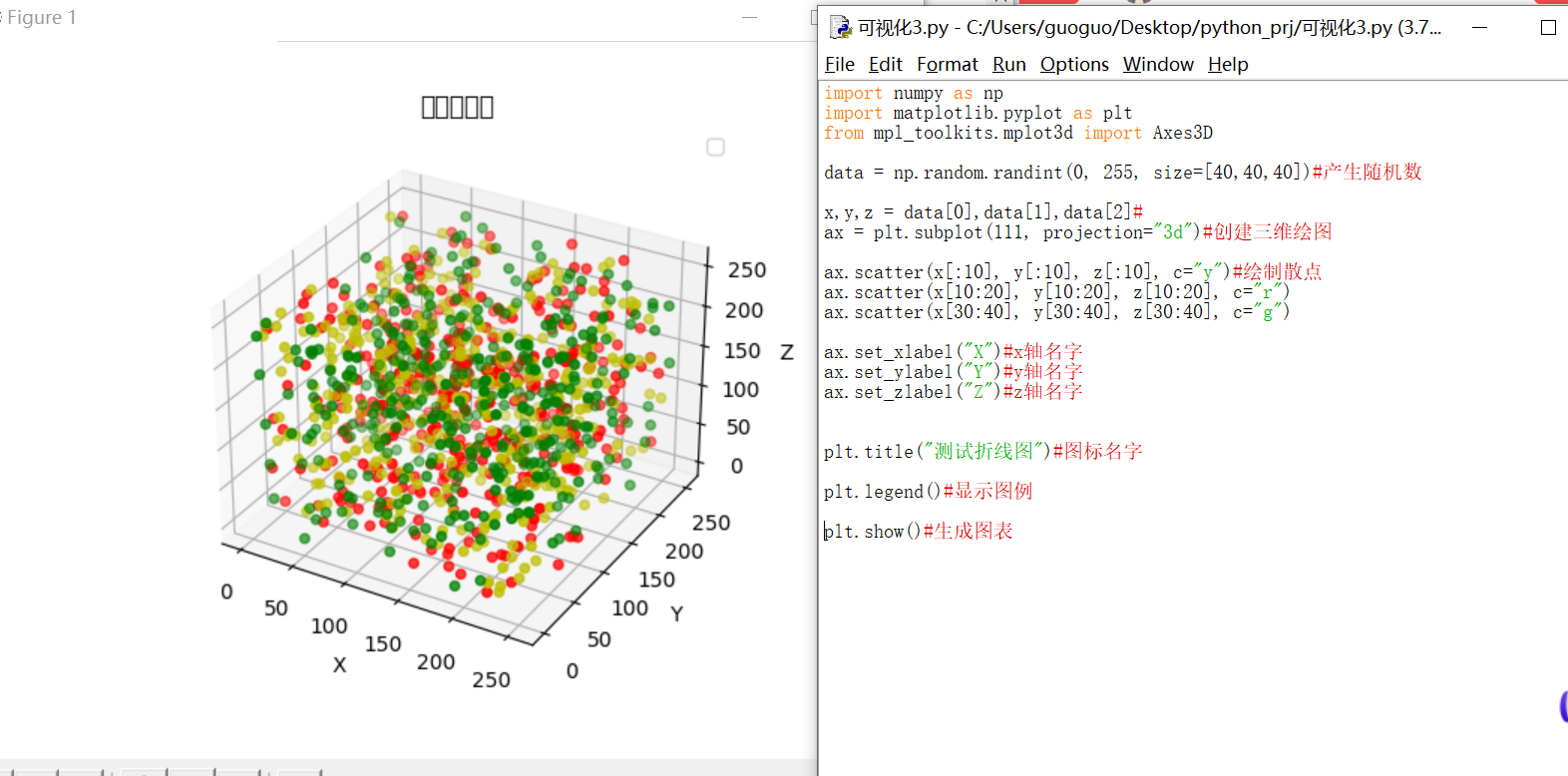五、三维平面图
import numpy as np
import matplotlib.pyplot as plt
from mpl_toolkits.mplot3d import Axes3D

fig = plt.figure()#创建一个新的画板
ax = Axes3D(fig)
X = np.arange(-4, 4, 0.25)#产生-4~4之间的等差数列，公差0.25
Y = np.arange(-4, 4, 0.25)
X,Y = np.meshgrid(X, Y)#画网格
R = np.sqrt(X**2 + Y**2)
Z = np.sin(R)#产生z值
ax.plot_surface(X, Y, Z, rstride=1, cstride=1, cmap="rainbow")#绘图

plt.show()#生成图表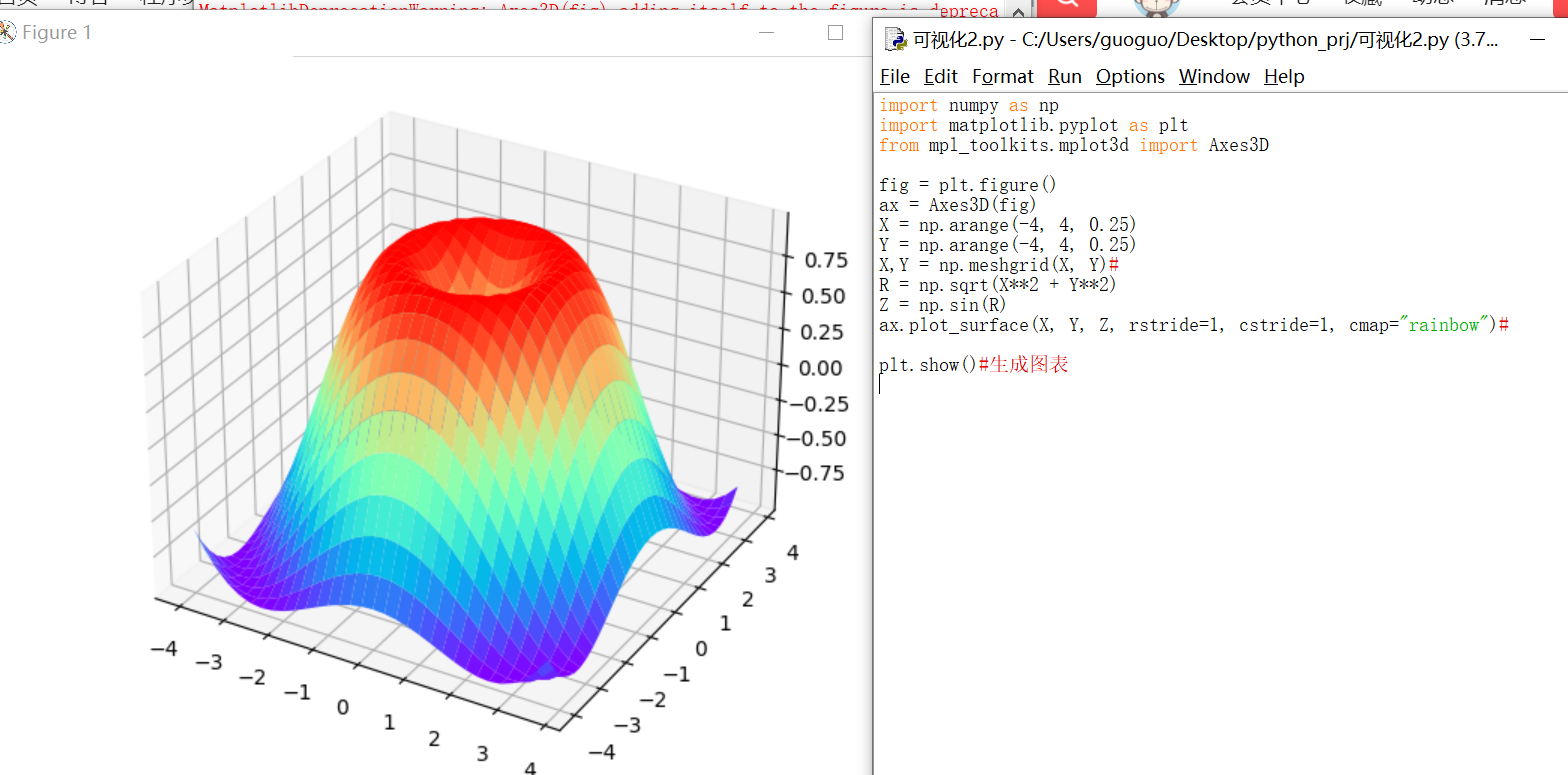展开全文数据分析
• 文章目录1、简介2、用 matplotlib 绘制各种可视化图表2.1 折线图2.2 柱形图2.3 饼图2.4 绘制三维散点图2.5 散点图3、其它可视化库3.1 Seaborn3.2 ggplot3.3 Bokeh3.4 pygal3.5 Plotly3.6 geoplotlib3.7 Gleam3.8 ...


文章目录
1、简介2、用 matplotlib 绘制各种可视化图表2.1 折线图2.2 柱形图2.3 饼图2.4 三维散点图2.5 散点图
3、其它可视化库3.1 Seaborn3.2 ggplot3.3 Bokeh3.4 pygal3.5 Plotly3.6 geoplotlib3.7 Gleam3.8 missingno3.9 Leather3.10 pastalog3.11 GazeParser
4、此项目Github源码分享

1、简介
最近项目中用到不少有关Python图表的知识，主要用的可视化程序库的泰斗 matplotlib 。其实还有很多相关画图表的图形库，大多数可视化图形库是基于 matplotlib 构建的并且确保一些用例更简单。本文主要讲的是 matplotlib ，在文尾将会介绍 11 种其它的数据可视化库。
matplotlib 是Python可视化程序库的泰斗。经过十几年它任然是Python使用者最常用的画图库。它的设计和在1980年代被设计的商业化程序语言MATLAB非常接近。
由于 matplotlib 是第一个 Python 可视化程序库，有许多别的程序库都是建立在它的基础上或者直接调用它。比如pandas和Seaborn就是matplotlib的外包，它们让你能用更少的代码去调用 matplotlib的方法。
虽然用matplotlib可以很方便的得到数据的大致信息，但是如果要更快捷简单地制作可供发表的图表就不那么容易了。就像Chris Moffitt 在“Python可视化工具简介”中提到的一样：“功能非常强大，也非常复杂。”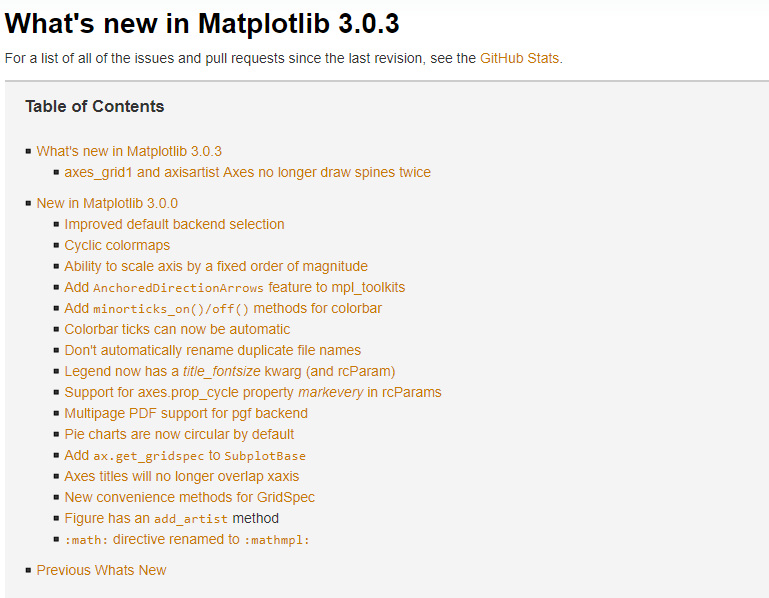官网：https://matplotlib.org/ Github/matplotlib：https://github.com/matplotlib/matplotlib
安装：
pip install matplotlib

2、用 matplotlib 绘制各种可视化图表
2.1 折线图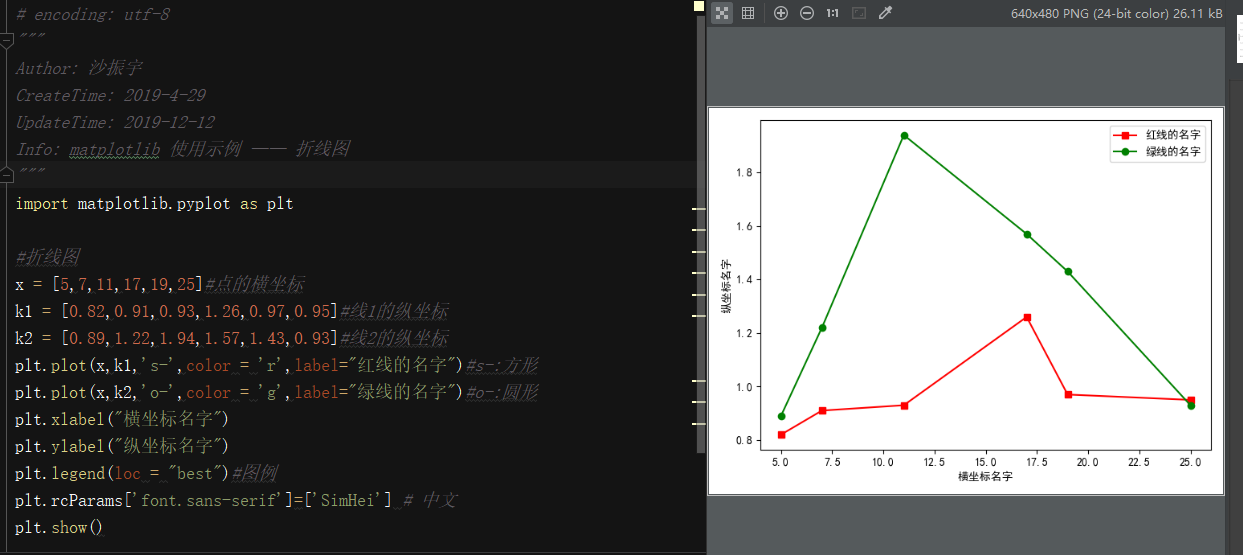# encoding: utf-8
"""
Author: 沙振宇
CreateTime: 2019-4-29
UpdateTime: 2019-12-12
Info: matplotlib 使用示例 —— 折线图
"""
import matplotlib.pyplot as plt

#折线图
x = [5,7,11,17,19,25]#点的横坐标
k1 = [0.82,0.91,0.93,1.26,0.97,0.95]#线1的纵坐标
k2 = [0.89,1.22,1.94,1.57,1.43,0.93]#线2的纵坐标
plt.plot(x,k1,'s-',color = 'r',label="红线的名字")#s-:方形
plt.plot(x,k2,'o-',color = 'g',label="绿线的名字")#o-:圆形
plt.xlabel("横坐标名字")
plt.ylabel("纵坐标名字")
plt.legend(loc = "best")#图例
plt.rcParams['font.sans-serif']=['SimHei'] # 中文
plt.show()

2.2 柱形图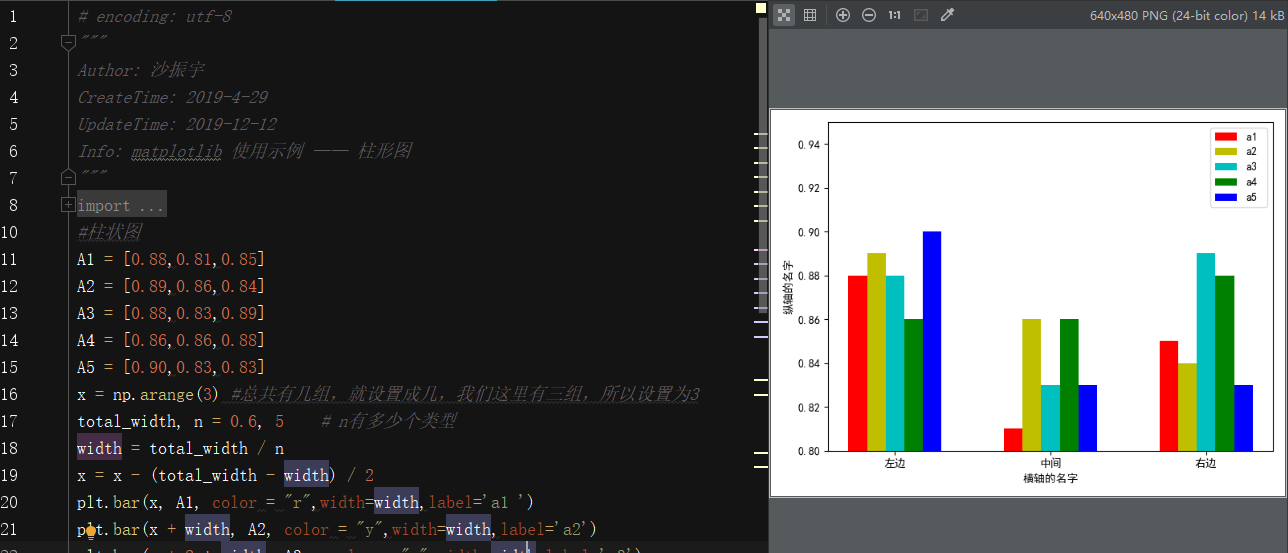# encoding: utf-8
"""
Author: 沙振宇
CreateTime: 2019-4-29
UpdateTime: 2019-12-12
Info: matplotlib 使用示例 —— 柱形图
"""
import numpy as np
import matplotlib.pyplot as plt
#柱状图
A1 = [0.88,0.81,0.85]
A2 = [0.89,0.86,0.84]
A3 = [0.88,0.83,0.89]
A4 = [0.86,0.86,0.88]
A5 = [0.90,0.83,0.83]
x = np.arange(3) #总共有几组，就设置成几，我们这里有三组，所以设置为3
total_width, n = 0.6, 5    # n有多少个类型
width = total_width / n
x = x - (total_width - width) / 2
plt.bar(x, A1, color = "r",width=width,label='a1 ')
plt.bar(x + width, A2, color = "y",width=width,label='a2')
plt.bar(x + 2 * width, A3 , color = "c",width=width,label='a3')
plt.bar(x + 3 * width, A4 , color = "g",width=width,label='a4')
plt.bar(x + 4 * width, A5 , color = "b",width=width,label='a5')
plt.xlabel("横轴的名字")
plt.ylabel("纵轴的名字")
plt.legend(loc = "best")
plt.xticks([0,1,2],['左边','中间','右边'])
plt.ylim((0.8, 0.95))
my_y_ticks = np.arange(0.8, 0.95, 0.02)
plt.yticks(my_y_ticks)
plt.rcParams['font.sans-serif']=['SimHei']  # 中文
plt.show()

2.3 饼图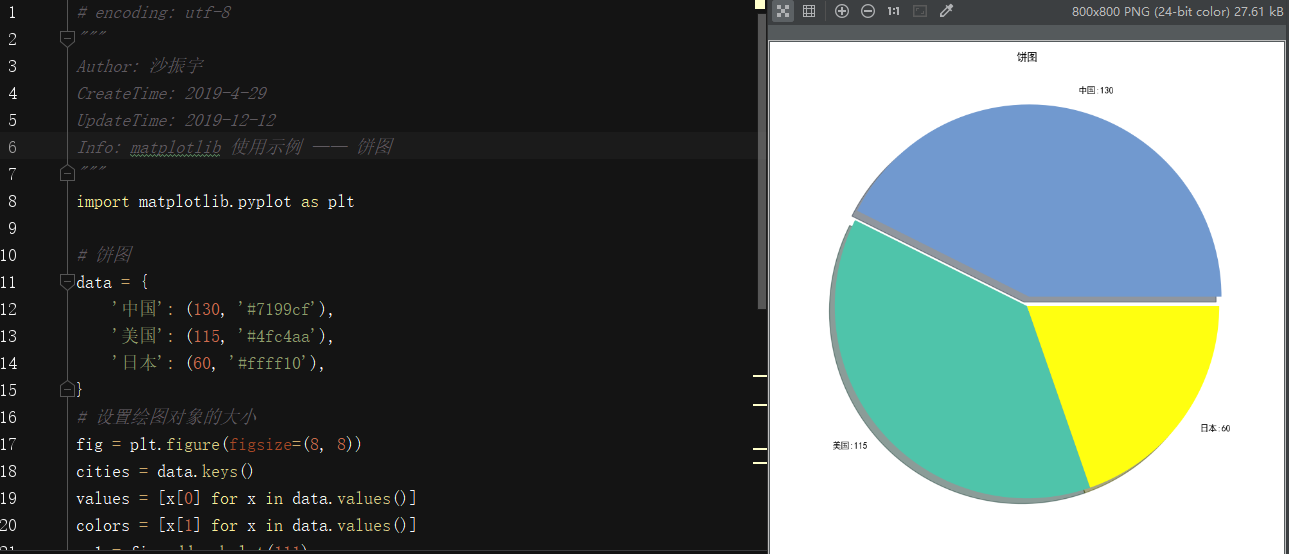# encoding: utf-8
"""
Author: 沙振宇
CreateTime: 2019-4-29
UpdateTime: 2019-12-12
Info: matplotlib 使用示例 —— 饼图
"""
import matplotlib.pyplot as plt

# 饼图
data = {
'中国': (130, '#7199cf'),
'美国': (115, '#4fc4aa'),
'日本': (60, '#ffff10'),
}
# 设置绘图对象的大小
fig = plt.figure(figsize=(8, 8))
cities = data.keys()
values = [x for x in data.values()]
colors = [x for x in data.values()]
ax1.set_title('饼图')
labels = ['{}:{}'.format(city, value) for city, value in zip(cities, values)]

explode = [0.05, 0, 0] # 设置饼图的凸出显示
ax1.pie(values, labels=labels, colors=colors, explode=explode, shadow=True) # 画饼状图， 并且指定标签和对应的颜色  指定阴影效果

# plt.savefig('pie.jpg') # 保存成图片
plt.rcParams['font.sans-serif']=['SimHei']  # 中文
plt.show()

2.4 三维散点图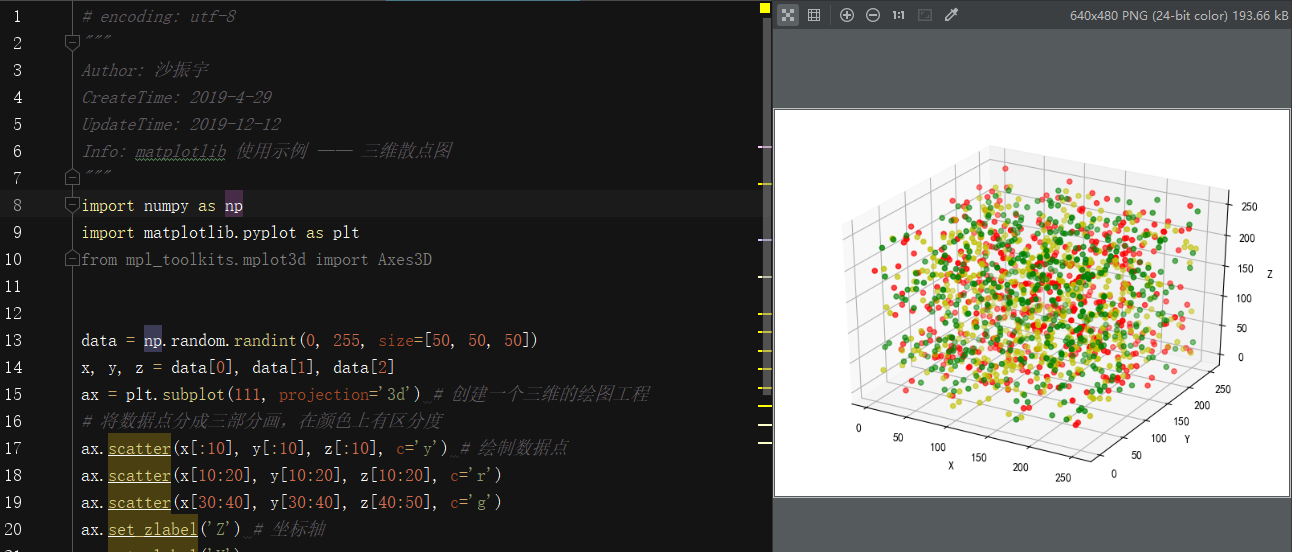# encoding: utf-8
"""
Author: 沙振宇
CreateTime: 2019-4-29
UpdateTime: 2019-12-12
Info: matplotlib 使用示例 —— 三维散点图
"""
import numpy as np
import matplotlib.pyplot as plt
from mpl_toolkits.mplot3d import Axes3D

data = np.random.randint(0, 255, size=[50, 50, 50])
x, y, z = data, data, data
ax = plt.subplot(111, projection='3d') # 创建一个三维的绘图工程
# 将数据点分成三部分画，在颜色上有区分度
ax.scatter(x[:10], y[:10], z[:10], c='y') # 绘制数据点
ax.scatter(x[10:20], y[10:20], z[10:20], c='r')
ax.scatter(x[30:40], y[30:40], z[40:50], c='g')
ax.set_zlabel('Z') # 坐标轴
ax.set_ylabel('Y')
ax.set_xlabel('X')
plt.rcParams['font.sans-serif']=['SimHei']  # 中文
plt.show()

2.5 散点图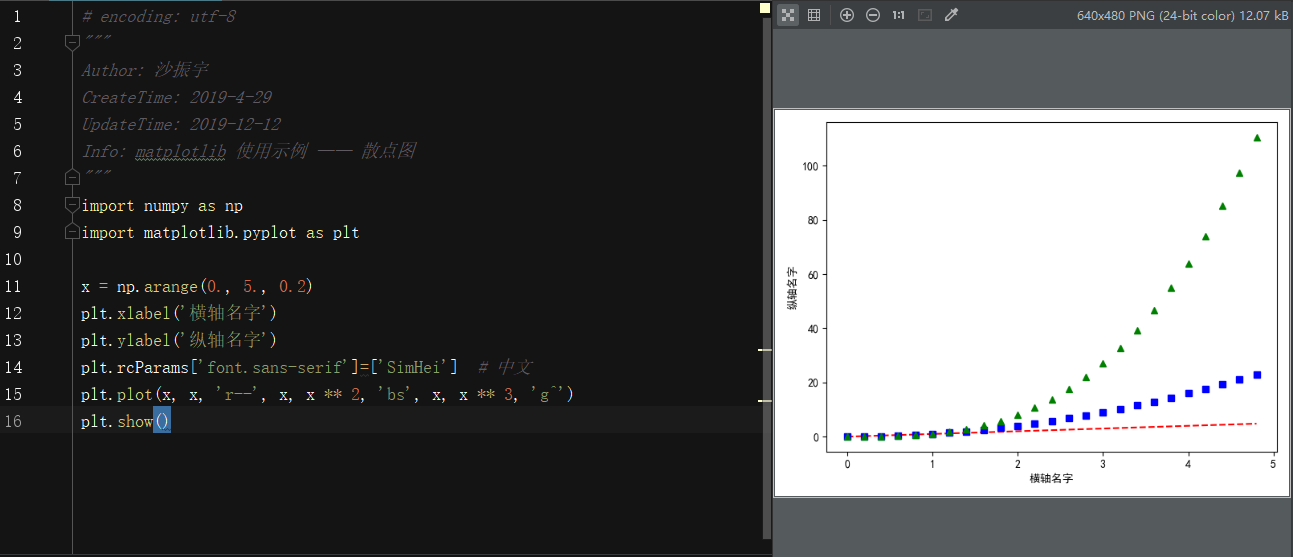# encoding: utf-8
"""
Author: 沙振宇
CreateTime: 2019-4-29
UpdateTime: 2019-12-12
Info: matplotlib 使用示例 —— 散点图
"""
import numpy as np
import matplotlib.pyplot as plt

x = np.arange(0., 5., 0.2)
plt.xlabel('横轴名字')
plt.ylabel('纵轴名字')
plt.rcParams['font.sans-serif']=['SimHei']  # 中文
plt.plot(x, x, 'r--', x, x ** 2, 'bs', x, x ** 3, 'g^')
plt.show()

3、其它可视化库
3.1 Seaborn
Seaborn利用了matplotlib，用简洁的代码来制作好看的图表。Seaborn跟matplotlib最大的区别就是它的默认绘图风格和色彩搭配都具有现代美感。由于Seaborn是构建在matplotlib的基础上的，你需要了解matplotlib从而来调整Seaborn的默认参数。 官网: http://seaborn.pydata.org/index.html
3.2 ggplot
ggplot 基于R的一个作图包 ggplot2, 同时利用了源于 《图像语法》（The Grammar of Graphics）中的概念。ggplot 跟 matplotlib 的不同之处是它允许你叠加不同的图层来完成一幅图。比如你可以从轴开始，然后加上点，加上线，趋势线等等。虽然《图像语法》得到了“接近思维过程”的作图方法的好评，但是习惯了matplotlib的用户可能需要一些时间来适应这个新思维方式。ggplot的作者提到 ggplot 并不适用于制作非常个性化的图像。它为了操作的简洁而牺牲了图像复杂度。ggplot跟pandas的整合度非常高，所以当你使用它的时候，最好将你的数据读成 DataFrame。 官网: http://ggplot.yhathq.com/
3.3 Bokeh
跟ggplot一样， Bokeh 也是基于《图形语法》的概念。但是跟ggplot不一样的是，它完全基于Python而不是从R引用过来的。它的长处在于它能用于制作可交互，可直接用于网络的图表。图表可以输出为JSON对象，HTML文档或者可交互的网络应用。Boken也支持数据流和实时数据。 Bokeh为不同的用户提供了三种控制水平。最高的控制水平用于快速制图，主要用于制作常用图像， 例如柱状图，盒状图，直方图。中等控制水平跟matplotlib一样允许你控制图像的基本元素（例如分布图中的点）。最低的控制水平主要面向开发人员和软件工程师。它没有默认值，你得定义图表的每一个元素。 官网: https://bokeh.pydata.org/en/latest/
3.4 pygal
pygal 跟 Bokeh 和 Plotly 一样，提供可直接嵌入网络浏览器的可交互图像。跟其他两者的主要区别在于它可以将图表输出为SVG格式。如果你的数据量相对小，SVG就够用了。但是如果你有成百上千的数据点，SVG的渲染过程会变得很慢。由于所有的图表都被封装成了方法，而且默认的风格也很漂亮，用几行代码就可以很容易地制作出漂亮的图表。 官网: http://www.pygal.org/en/latest/index.html
3.5 Plotly
在线制图工具Plotly，但是你知道你可以通过Python notebook使用它么？Plotly 跟 Bokeh 一样致力于交互图表的制作，但是它提供在别的库中很难找到的几种图表类型，比如等值线图，树形图和三维图表。 官网: https://plot.ly/python/
3.6 geoplotlib
geoplotlib 是一个用于制作地图和地理相关数据的工具箱。你可以用它来制作多种地图，比如等值区域图， 热度图，点密度图。你必须安装 Pyglet （一个面向对象编程接口）来使用geoplotlib。 不过因为大部分Python的可视化工具不提供地图，有一个专职画地图的工具也是挺方便的。 Github:https://github.com/andrea-cuttone/geoplotlib
3.7 Gleam
Gleam 借用了R中 Shiny 的灵感。 它允许你只利用 Python 程序将你的分析变成可交互的网络应用，你不需要会用HTML CSS 或者 JaveScript。Gleam 可以使用任何一种 Python 的可视化库。当你创建一个图表的时候，你可以在上面加上一个域，这样用户可以用它来对数据排序和过滤了。 Github:https://github.com/dgrtwo/gleam
3.8 missingno
缺失数据是永远的痛。missingno 用图像的方式让你能够快速评估数据缺失的情况，而不是在数据表里面步履维艰。你可以根据数据的完整度对数据进行排序或过滤，或者根据热度图或树状图来考虑对数据进行修正。 Github: https://github.com/ResidentMario/missingno
3.9 Leather
3.10 pastalog
面向训练神经网络的简单实时可视化服务器。 Lasagne。Keras。Tensorflow。Torch。Theano和基本所有其他内容一起使用。 Github: https://github.com/rewonc/pastalog
3.11 GazeParser
GazeParser是眼动研究的一个包。 对于录制，该软件包提供了一个模块来控制来自VisionEgg和PsychoPy的基于视频的开源眼动追踪应用程序SimpleGazeTracker。 对于数据分析，该软件包提供各种功能，例如检测扫视和固定，绘制和比较扫描路径，计算扫视轨迹曲率等。 pypi：https://pypi.org/project/GazeParser/0.11.1/
4、此项目Github源码分享
https://github.com/ShaShiDiZhuanLan/Demo_Matplotlib_Python
展开全文matplotlib 折线图 饼图 柱形图
• 第一章内容发布在（使用Python制作疫情数据分析可视化图表（一））https://blog.csdn.net/yue__yang/article/details/104538235，请自行食用。 二、时间序列与区域划分 1、数据类型转换为时间序列 在数据中，有一个...
• 喜欢用 Python 做项目的小伙伴不免会遇到这种情况：做图表时，用哪种好看又实用的可视化工具包呢？今天小编给大家分享8个非常流行的 Python 可视化工具包,需要的朋友可以参考下
• Python可视化图表生成-Matplotlib Matplotlib 是Python中类似 MATLAB 的绘图工具，熟悉 MATLAB 也可以很快的上手 Matplotlib 安装 pip install matplotlib 折线图 import numpy as npimport matplotlib....
Python可视化图表生成-Matplotlib
Matplotlib 是Python中类似 MATLAB 的绘图工具，熟悉 MATLAB 也可以很快的上手 Matplotlib
安装

pip install matplotlib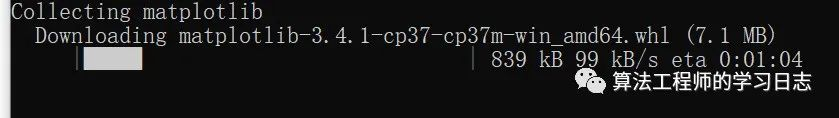折线图
import numpy as npimport matplotlib.pyplot as pltimport matplotlib
# 指定默认字体matplotlib.rcParams['font.sans-serif'] = ['SimHei']matplotlib.rcParams['font.family'] = 'sans-serif'# 解决负号'-'显示为方块的问题matplotlib.rcParams['axes.unicode_minus'] = False
x = np.linspace(0, 2, 100)  # 创建等差数列 0-2之间100个
plt.plot(x, x, label="line1")  # 第一个参数为横坐标 第二个为纵坐标 第三个为曲线名字plt.plot(x, x ** 2, label="line2")plt.plot(x, x ** 3, label="line3")plt.xlabel("x label")  # x轴名字plt.ylabel("y label")  # y轴名字plt.title("折线图")  # 图标名字plt.legend()  # 显示图例plt.show()  # 生成图表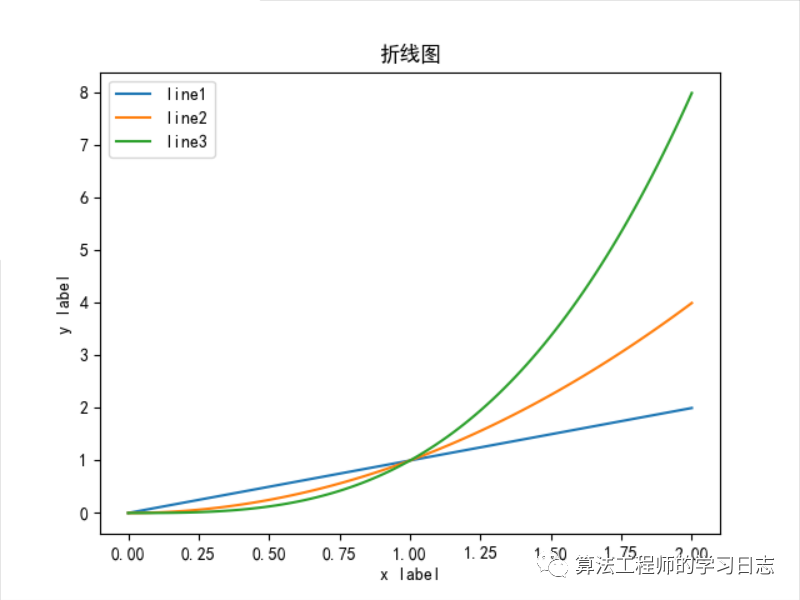散点图
​​​​​​​
import numpy as npimport matplotlib.pyplot as pltimport matplotlib
# 指定默认字体matplotlib.rcParams['font.sans-serif'] = ['SimHei']matplotlib.rcParams['font.family'] = 'sans-serif'# 解决负号'-'显示为方块的问题matplotlib.rcParams['axes.unicode_minus'] = False
x = np.arange(0., 5., 0.2)  # 从0-5之间，产生等差数列，公差为0.2plt.plot(x, x, "r--", x, x ** 2, "bs", x, x ** 3, "g^")  #plt.xlabel("x label")  # x轴名字plt.ylabel("y label")  # y轴名字plt.title("折线图")  # 图标名字plt.legend()  # 显示图例
plt.show()  # 生成图表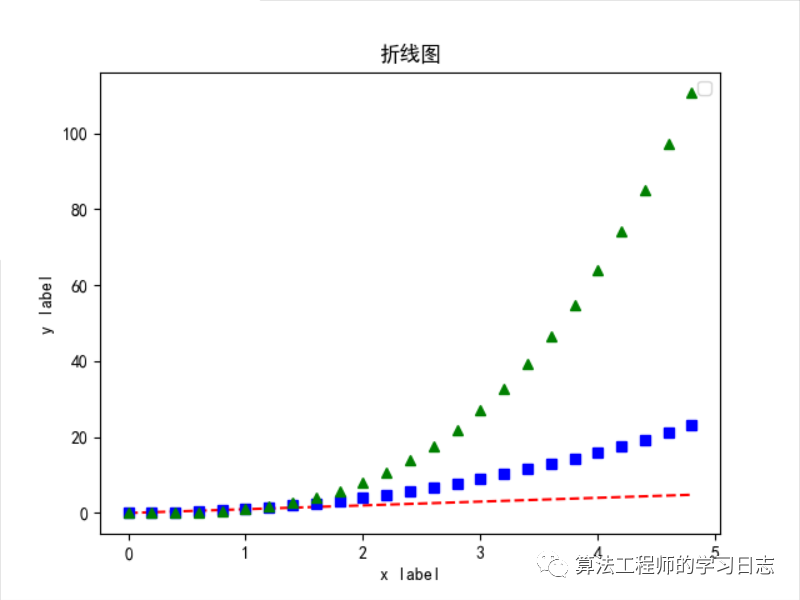三维散点图
​​​​​​​
import numpy as npimport matplotlib.pyplot as pltimport matplotlib
# 指定默认字体matplotlib.rcParams['font.sans-serif'] = ['SimHei']matplotlib.rcParams['font.family'] = 'sans-serif'# 解决负号'-'显示为方块的问题matplotlib.rcParams['axes.unicode_minus'] = False
data = np.random.randint(0, 255, size=[40, 40, 40])  # 产生随机数
x, y, z = data, data, data  #ax = plt.subplot(111, projection="3d")  # 创建三维绘图
ax.scatter(x[:10], y[:10], z[:10], c="y")  # 绘制散点ax.scatter(x[10:20], y[10:20], z[10:20], c="r")ax.scatter(x[30:40], y[30:40], z[30:40], c="g")
ax.set_xlabel("X")  # x轴名字ax.set_ylabel("Y")  # y轴名字ax.set_zlabel("Z")  # z轴名字
plt.title("三维散点图")  # 图标名字plt.legend()  # 显示图例plt.show()  # 生成图表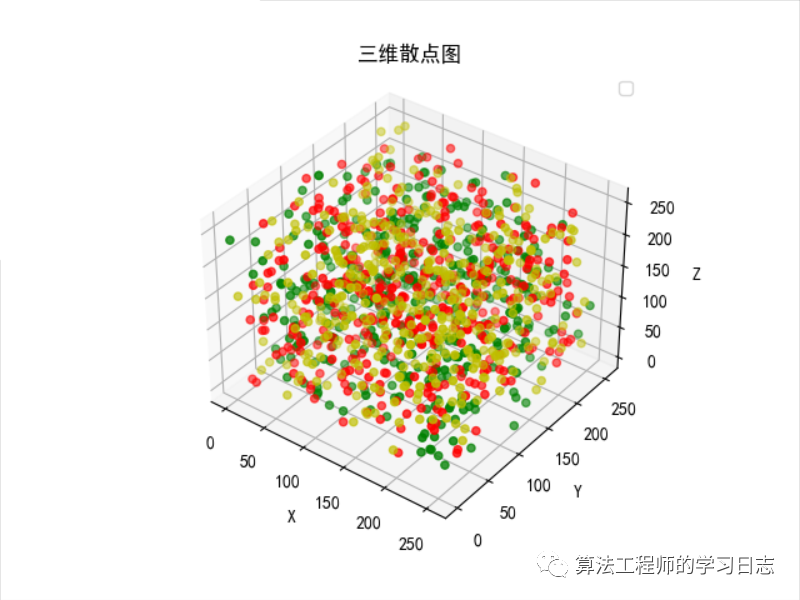三维平面图
import numpy as npimport matplotlib.pyplot as pltimport matplotlibfrom mpl_toolkits.mplot3d import Axes3D
# 指定默认字体matplotlib.rcParams['font.sans-serif'] = ['SimHei']matplotlib.rcParams['font.family'] = 'sans-serif'# 解决负号'-'显示为方块的问题matplotlib.rcParams['axes.unicode_minus'] = False
fig = plt.figure()ax = Axes3D(fig)X = np.arange(-4, 4, 0.25)Y = np.arange(-4, 4, 0.25)X, Y = np.meshgrid(X, Y)  #R = np.sqrt(X ** 2 + Y ** 2)Z = np.sin(R)ax.plot_surface(X, Y, Z, rstride=1, cstride=1, cmap="rainbow")  #
plt.show()  # 生成图表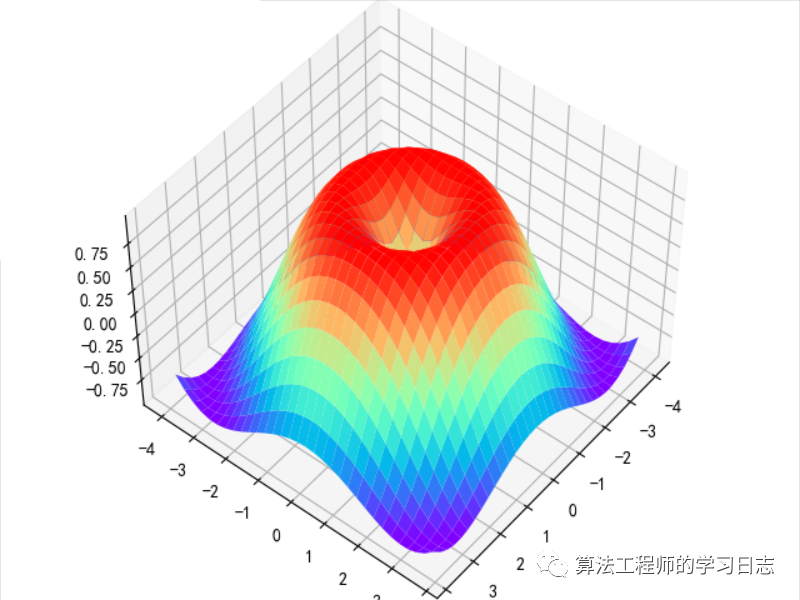展开全文• 主要给大家介绍了关于Python数据可视化教程之利用Matplotlib实现各种图表的相关资料，文中通过示例代码介绍的非常详细，对大家的学习或者工作具有一定的参考学习价值，需要的朋友们下面来一起看看吧
• 可视化图表，有相当多种，但常见的也就下面几种，其他比较复杂一点，大都也是基于如下几种进行组合，变换出来的。对于初学者来说，很容易被这官网上众多的图表类型给吓着了，由于种类太多，几种图表的绘制方法很有...
前言
本文的文字及图片来源于网络,仅供学习、交流使用,不具有任何商业用途,如有问题请及时联系我们以作处理。
PS：如有需要Python学习资料的小伙伴可以加点击下方链接自行获取
python免费学习资料以及群交流解答点击即可加入
可视化图表，有相当多种，但常见的也就下面几种，其他比较复杂一点，大都也是基于如下几种进行组合，变换出来的。对于初学者来说，很容易被这官网上众多的图表类型给吓着了，由于种类太多，几种图表的绘制方法很有可能会混淆起来。
因此，在这里，我特地总结了六种常见的基本图表类型，你可以通过对比学习，打下坚实的基础。
01. 折线图
绘制折线图，如果你数据不是很多的话，画出来的图将是曲折状态，但一旦你的数据集大起来，比如下面我们的示例，有100个点，所以我们用肉眼看到的将是一条平滑的曲线。
这里我绘制三条线，只要执行三次plt.plot就可以了。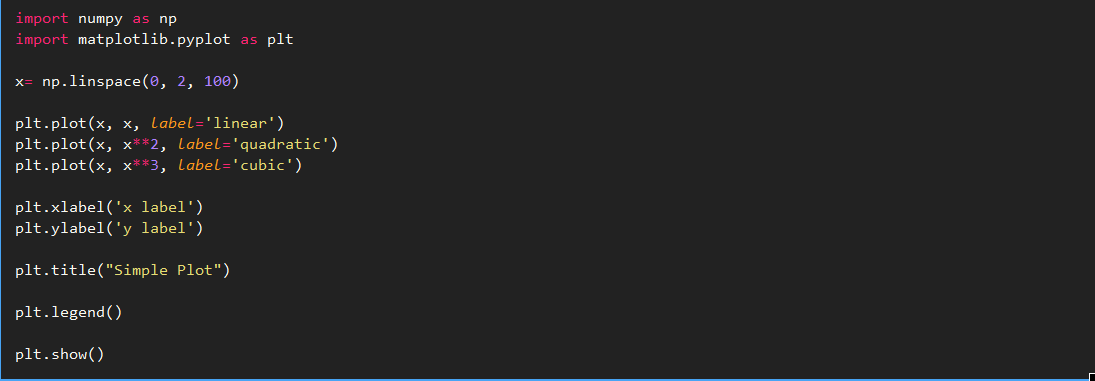show image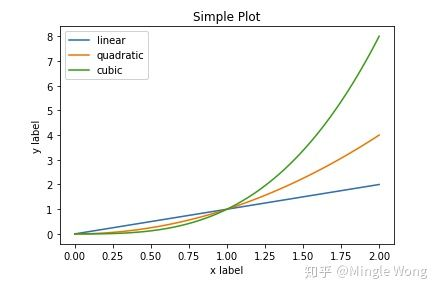02. 散点图
其实散点图和折线图是一样的原理，将散点图里的点用线连接起来就是折线图了。所以绘制散点图，只要设置一下线型即可。
注意：这里我也绘制三条线，和上面不同的是，我只用一个plt.plot就可以了。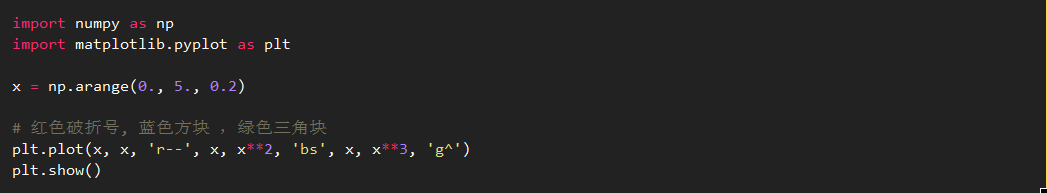show image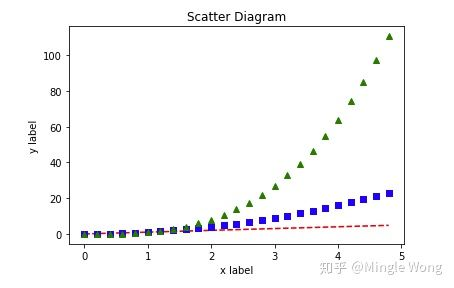03. 直方图
直方图，大家也不算陌生了。这里小明加大难度，在一张图里，画出两个频度直方图。这应该在实际场景上也会遇到吧，因为这样真的很方便比较，有木有？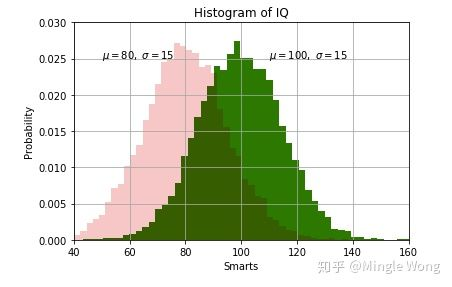04. 柱状图
同样的，简单的柱状图，我就不画了，这里画三种比较难的图。
4.1 并列柱状图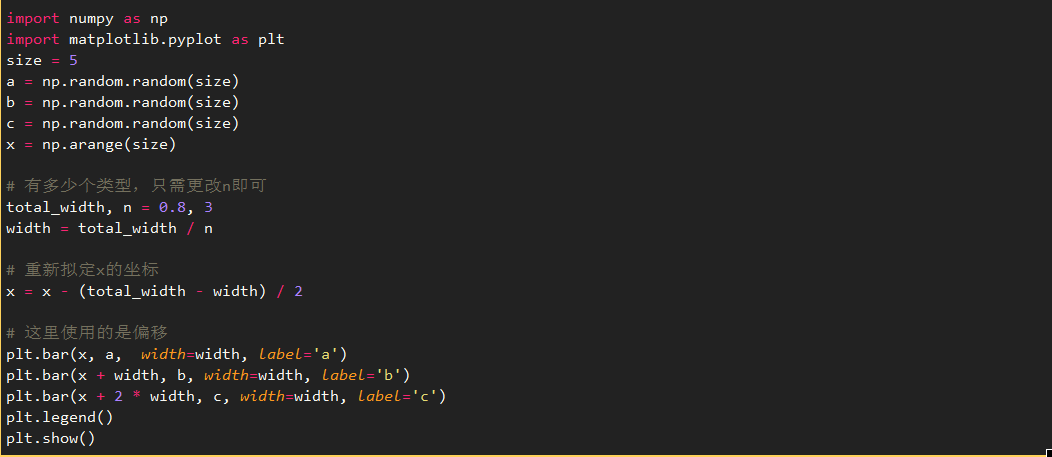show image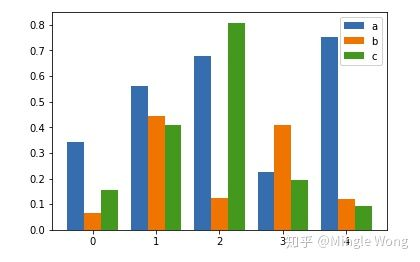4.2 叠加柱状图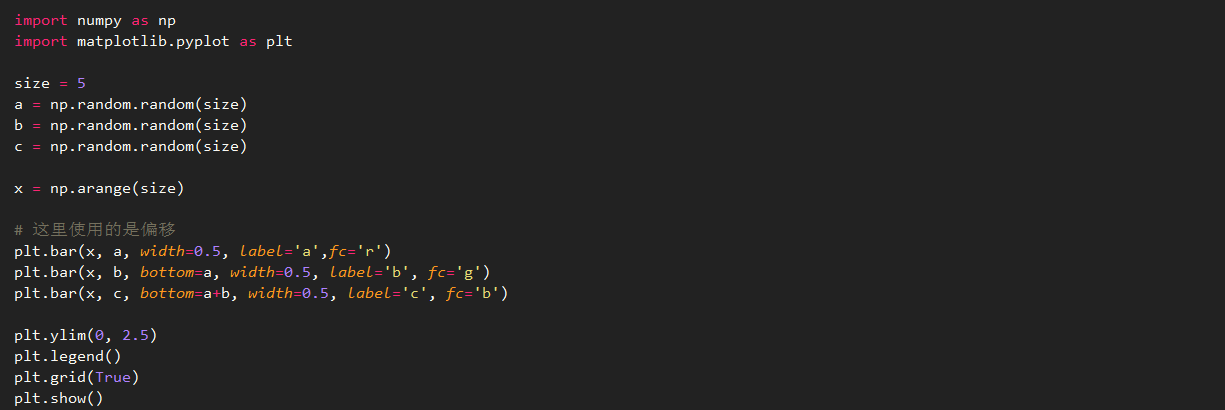show image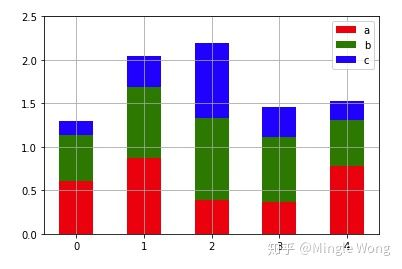05. 饼图
5.1 普通饼图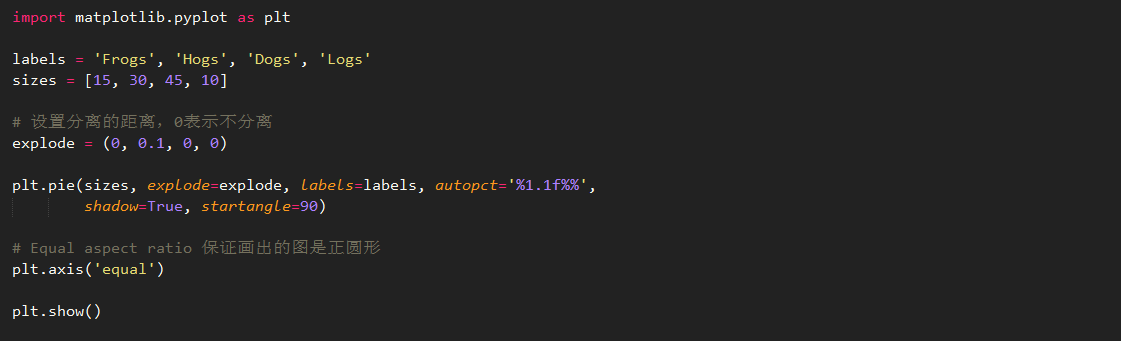show image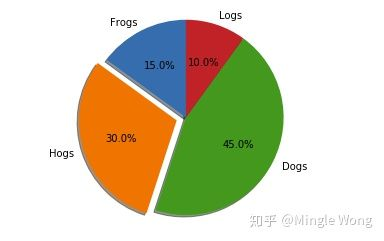5.2 嵌套饼图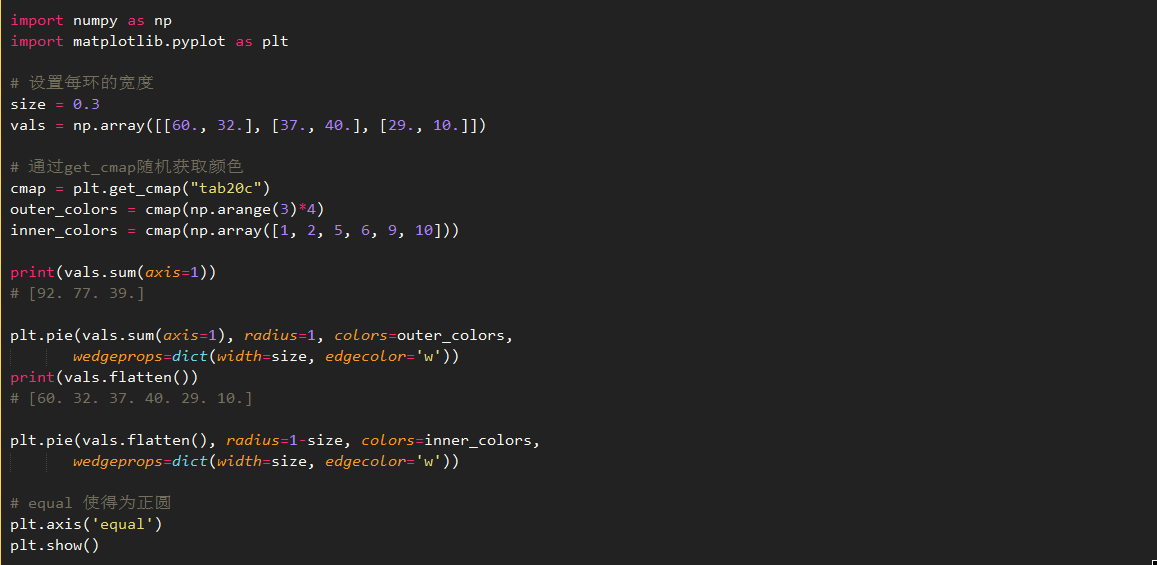show image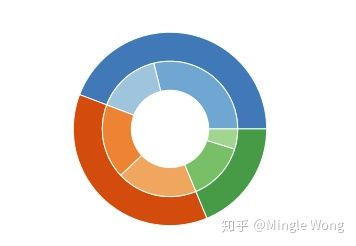5.3 极轴饼图
要说酷炫，极轴饼图也是数一数二的了，这里肯定也要学一下。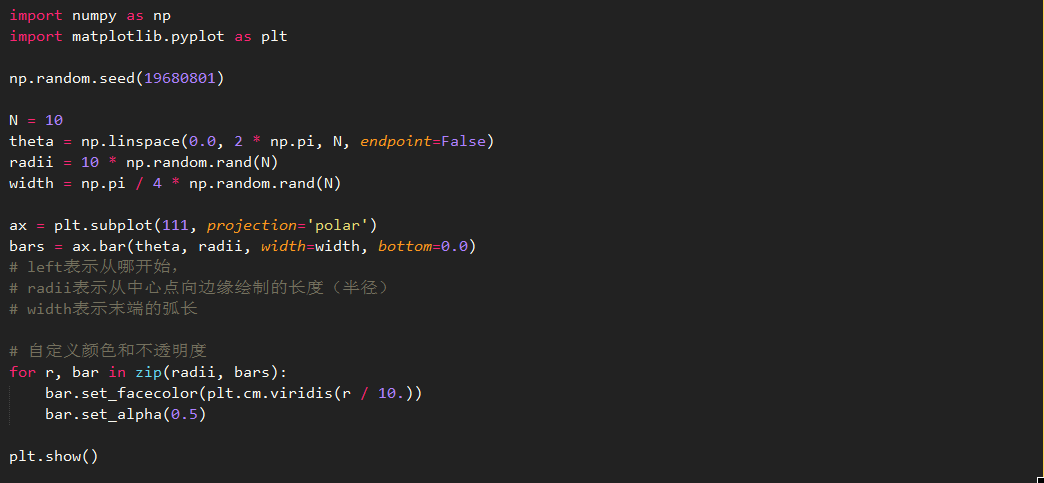show image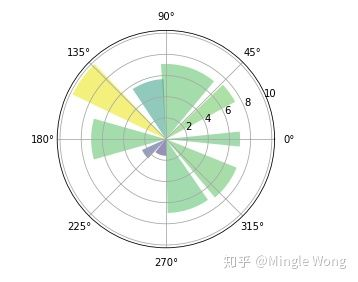06. 三维图
6.1 绘制三维散点图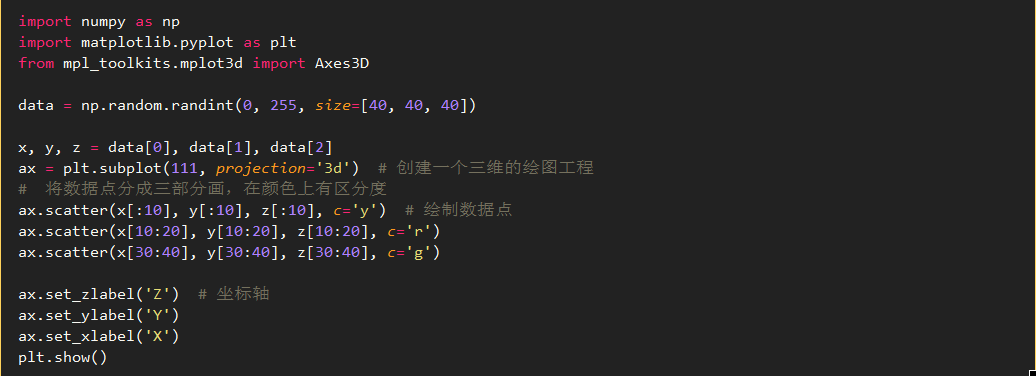show image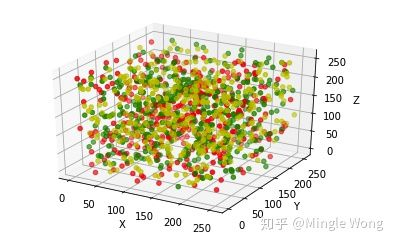6.2 绘制三维平面图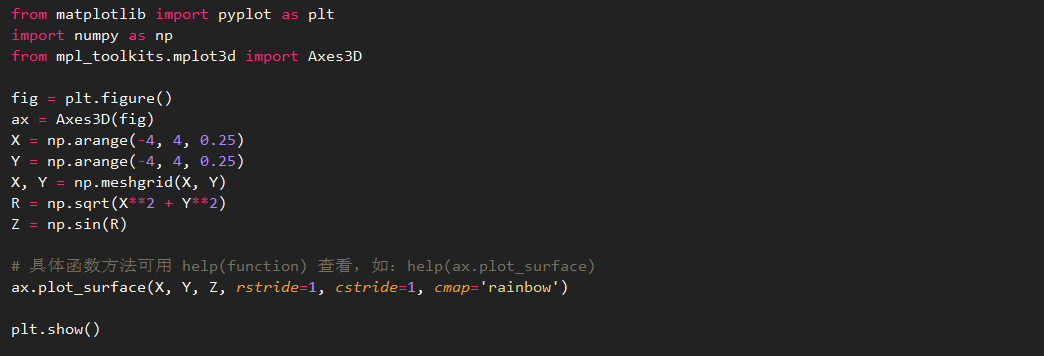show image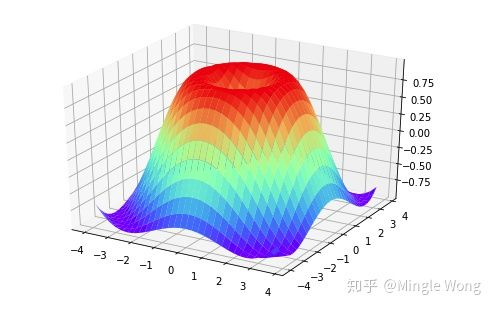展开全文• 对于人类来说，图案和文字这两种信息表现方式，图案应该更早于文字被发明，现代考古人员时常在...今天我们就来讲讲在Python中如何把数据转换为可视化图表。 本文知识点： pyecharts简介与安装 pyecharts创建第...
• ## python可视化图表生成（二）

千次阅读 多人点赞 2021-04-28 20:10:42
plt.grid(True)#显示网格线 plt.show()#生成图表 2、叠加 import numpy as np import matplotlib.pyplot as plt size = 5 a = np.random.random(size)#产生5个随机数 b = np.random.random(size) c = np.random....
• Python使用matplotlib可视化绘制并导出可视化结果图表到PDF文件中 #仿真数据1 from pandas import DataFrame Data1 = {'Unemployment_Rate': [6.1,5.8,5.7,5.7,5.8,5.6,5.5,5.3,5.2,5.2], 'Stock_Index_Price...数据挖掘 机器学习 人工智能 自然语言处理
• 绘制常用图表折线图柱形图普通柱形图簇状柱形图堆积柱形图条形图散点图 折线图 plt.plot(x,y,color,linestyle,linewidth,marker,markeredecolor,markeregwidth,markerfacecolor,markersize,lable) x,y表示x轴...Matplotlib
• How the latest Python-PowerBI integration can be used to leverage the advanced visuals from python combined with the native data drill through and DAX functionalities 如何使用最新的Python-PowerBI集成...人工智能
• 今天小编就为大家分享一篇Python数据分析:手把手教你用Pandas生成可视化图表的教程，具有很好的参考价值，希望对大家有所帮助。一起跟随小编过来看看吧
• Python数据可视化matplotlib（一）—— 图表的基本元素 图表创建 plt.show() # 图表窗口1 → plt.show() plt.plot(np.random.rand(10)) plt.show() # 直接生成图表 魔法函数 % matplotlib inline ...matplotlib
• 如何才能在短时间内制作漂亮的可视化地图呢，我觉得Python+可视化工具是不错的选择。 以下动态可视化地图就是J哥亲手绘制，展现了一段时间内广州市企事业单位在网上商城采购商品的分布及随时间的变化。 接下来，将...
• 喜欢用 Python 做项目的小伙伴不免会遇到这种情况：做图表时，用哪种好看又实用的可视化工具包呢？之前文章里出现过漂亮的图表时，也总有读者在后台留言问该图表时用什么工具做的。下面，作者介绍了八种在 Python 中...
• 在数据分析与机器学习中，我们经常要用到大量的可视化操作。一张制作精美的数据图片，不仅能展示大量的信息，更能快速传达作者的想法、态度、水平、以及审美等素质...
• 今天小编就为大家分享一篇Python数据可视化:饼状图的实例讲解，具有很好的参考价值，希望对大家有所帮助。一起跟随小编过来看看吧
• json数据转 csv import json import time import csv file = open('DXYArea-TimeSeries.json','r',encoding='utf-8') infos = json.load(file) with open('data.csv','a',newline='') as f: writer = csv....
• 项目实现知识点： Pandas库及pyecharts库...Python数据可视化：漏斗图的制作 项目实现过程： 1.导入模块 2.打开文件 3.读取数据 4.整理数据 5.创建漏斗图 6.添加组件 7.显示漏斗并设置名称 8.结果展示...

# python可视化图表python 订阅